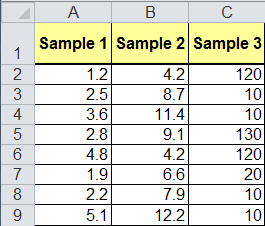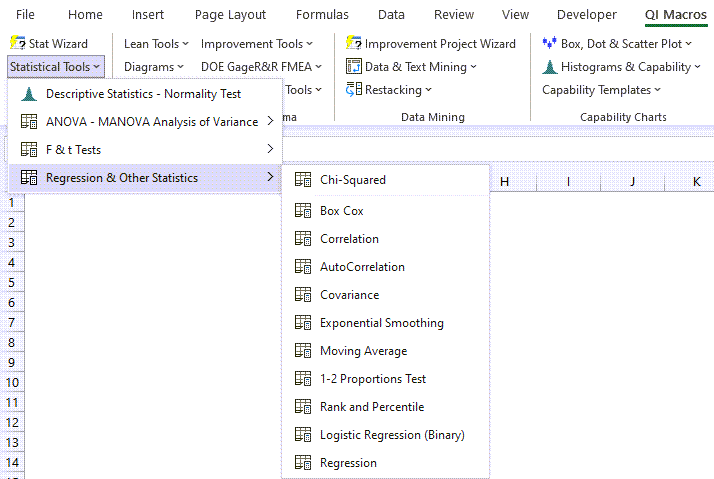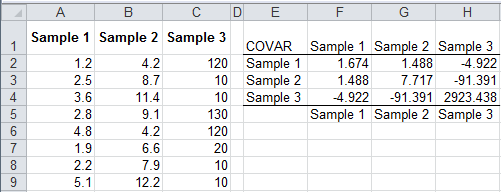# Covariance Example in Excel

## What is Covariance?

Imagine that you'd like to know if variation in one variable is related to the variation in another. While statistical analysis cannot prove that one thing causes another, it can determine if there is a relationship between the variables which gives a direction to the analysis.

Covariance: To understand and explore the relationships between two or more sets of numbers, QI Macros provides tools to analyze the variance (i.e., co-variance) and relationships (i.e., co-relation) between two sets of numbers.

### Systolic Blood Pressure vs Weight Covariance Example

This example uses QI Macros add-in for Excel, which is much easier to use than Excel's data analysis toolpak.

#### 1. Select the data

Select two or more columns of data:#### 2. Click on QI Macros menu, Statistical Tools, Regression and Other Statistics, then Covariance:#### 3. Evaluate the Covariance Results:Two variables can be positively correlated (more of one means more of another) or negatively correlated (more of one means less of another).

### Why Choose QI Macros Statistical Software for Excel?### Affordable

• Only \$329 USD - less with quantity discounts
• No annual fees
• Free Technical Support### Easy to Use

• Works Right in Excel
• Interprets Results for You
• Accurate Results Without Worry### Proven and Trusted

• 100,000 Users in 80 Countries
• Celebrating 20th Anniversary
• Five Star CNET Rating - Virus Free